Learning Library
Who we are
Guidance

# 4.NBT.B.5 Worksheets, Workbooks, Lesson Plans, and Games

#### CCSS.MATH.CONTENT.4.NBT.B.5

:
"Multiply a whole number of up to four digits by a one-digit whole number, and multiply two two-digit numbers, using strategies based on place value and the properties of operations. Illustrate and explain the calculation by using equations, rectangular arrays, and/or area models."

These worksheets, lesson plans, and exercises can help students practice this Common Core State Standards skill.

## Worksheets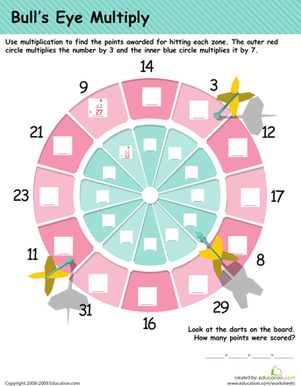Multiplication Practice: Darts!
Worksheet
Multiplication Practice: Darts!
In this 4th grade math worksheet, your child will practice his multiplication tables as he calculates the points on a dart board.
Math
Worksheet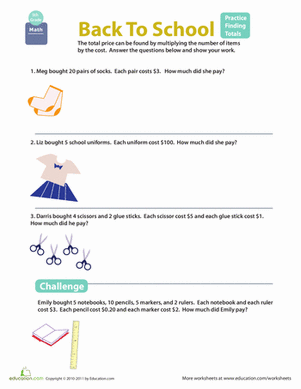Totaling Practice
Worksheet
Totaling Practice
Can your child help determine the total prices to be paid for back-to-school goods? She'll use her multiplication skills to help her out.
Math
Worksheet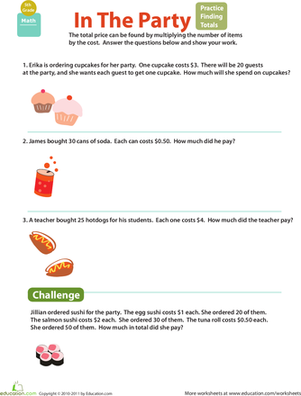Money Math: Practice Finding Totals
Worksheet
Money Math: Practice Finding Totals
Can your fifth grader ring up the total prices? She'll use her multiplication muscles in the process!
Math
Worksheet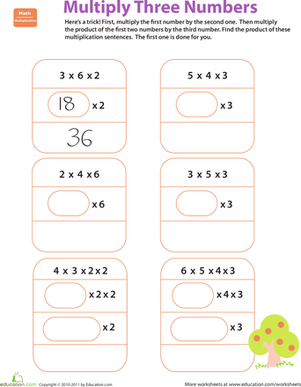Quick Trick: Multiply Three Numbers
Worksheet
Quick Trick: Multiply Three Numbers
This valuable worksheet teaches your fourth grader how to multiply three numbers, and gives him practice with the skill.
Math
Worksheet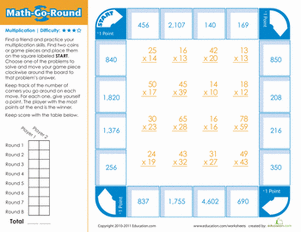Math-Go-Round: Hard
Worksheet
Math-Go-Round: Hard
Here's a fun board game that's sure to take the blahs out of multiplication practice.
Math
Worksheet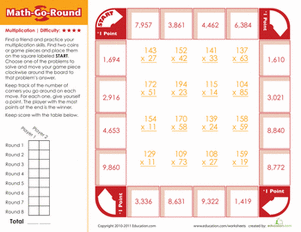Math-Go-Round: Expert
Worksheet
Math-Go-Round: Expert
Is your child an expert at multiplication? Find out with this fun, printable board game.
Math
Worksheet

## Lesson Plans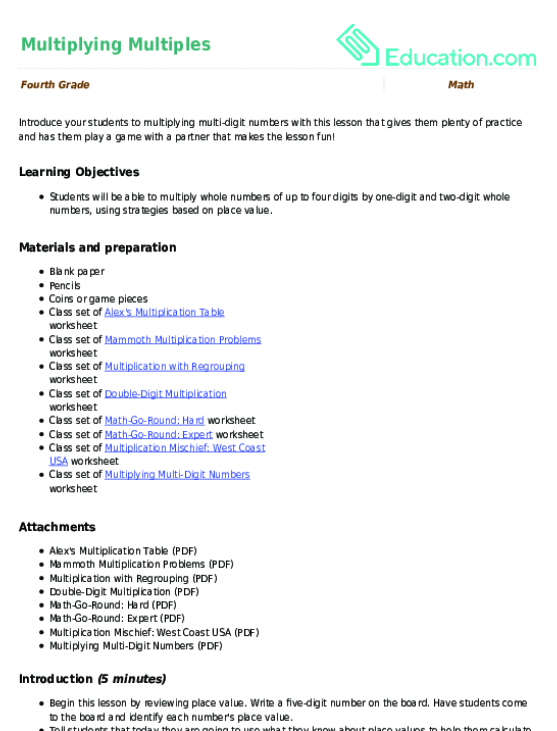Multiplying Multiples
Lesson plan
Multiplying Multiples
Introduce your students to multiplying multi-digit numbers with this lesson that gives them plenty of practice and has them play a game with a partner that makes the lesson fun!
Math
Lesson plan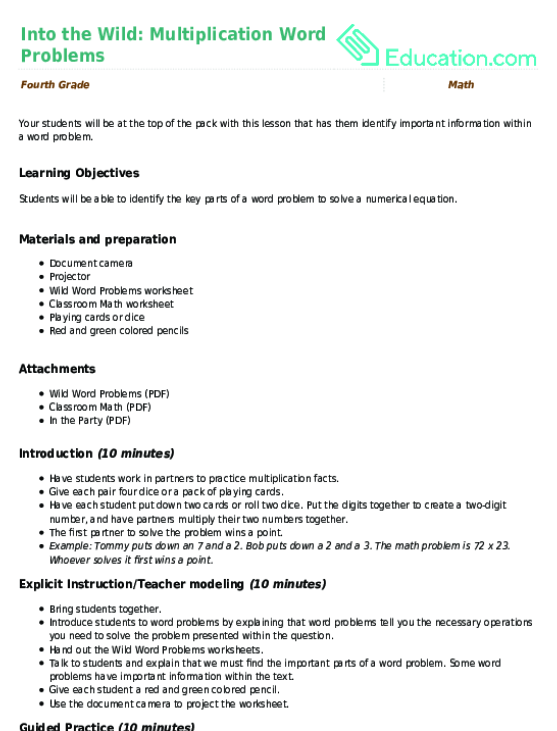Into the Wild: Multiplication Word Problems
Lesson plan
Into the Wild: Multiplication Word Problems
Your students will be at the top of the pack with this lesson that has them identify important information within a word problem.
Math
Lesson planMultiplication Bingo: Two-Digit Numbers
Lesson plan
Multiplication Bingo: Two-Digit Numbers
Your students will go bonkers for bingo as they play this baffling multiplication game! Who will get five in a row first?
Math
Lesson planProblems with Parentheses
Lesson plan
Problems with Parentheses
Students will explore how parentheses change the meaning of a number sentence. They will be challenged with a set of playing cards to make a certain number.
Math
Lesson plan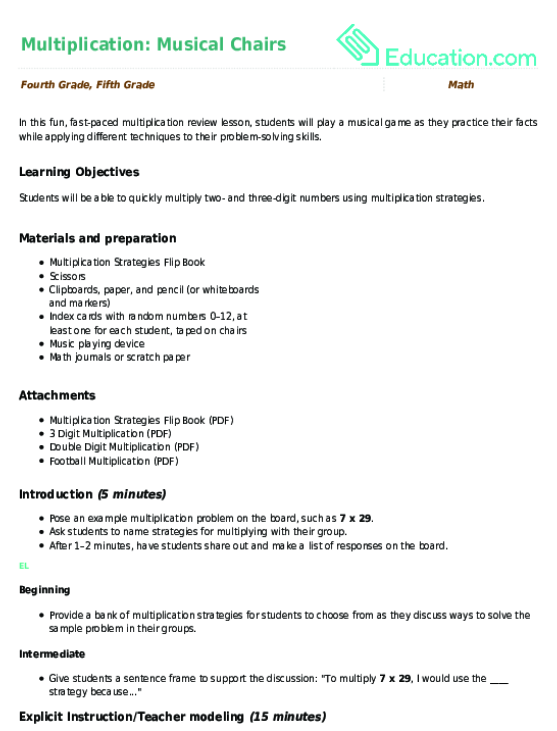Multiplication: Musical Chairs
Lesson plan
Multiplication: Musical Chairs
In this fun, fast-paced multiplication review lesson, students will play a musical game as they practice their facts while applying different techniques to their problem-solving skills.
Math
Lesson planArranging Numbers in Multiple Ways
Lesson plan
Arranging Numbers in Multiple Ways
In this lesson, students use cubes to build arrays to represent factor pairs of numbers within 100. They use this understanding to identify multiples as well.
Math
Lesson plan

## Workbooks

No workbooks found for this common core node.

## Games

No games found for this common core node.

## ExercisesMulti-Digit Multiplication and Doubling
Exercise
Multi-Digit Multiplication and Doubling
Aid your fourth grader in learning multi-digit multiplication with these exercises that show students how to visualize doubling.
Math
Exercise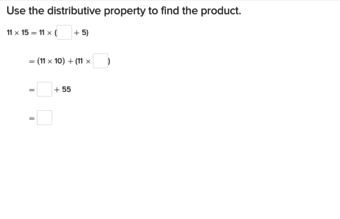Distributive Property of Multiplication
Exercise
Distributive Property of Multiplication
Help fourth graders solidify their knowledge of multiplication by teaching them the distributive property and how it can aid them in solving problems.
Math
Exercise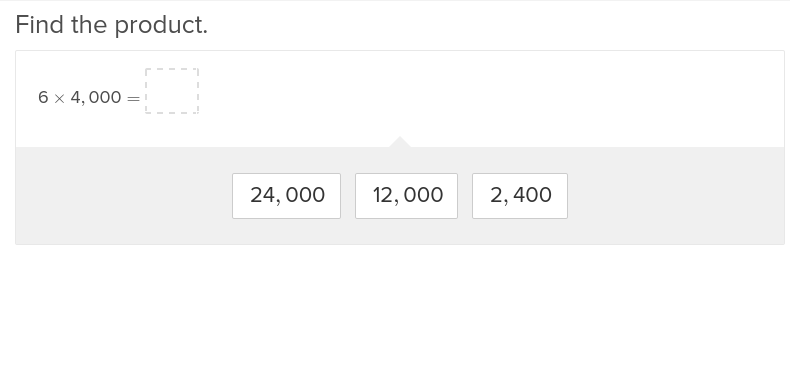Multiply by Multiples of Ten
Exercise
Multiply by Multiples of Ten
Teach fourth graders tricks and tips to multiplying by multiples of ten with these exercises that allow students to work independently.
Math
Exercise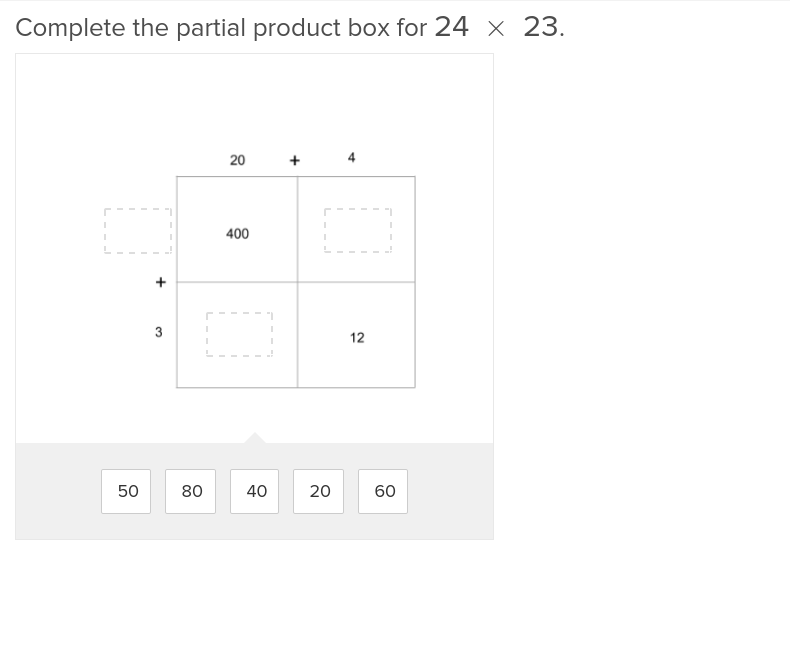Multi-Digit Multiplication and Partial Products 1
Exercise
Multi-Digit Multiplication and Partial Products 1
Break down multi-digit multiplication with these exercises that lead students step-by-step through the partial product method.
Math
Exercise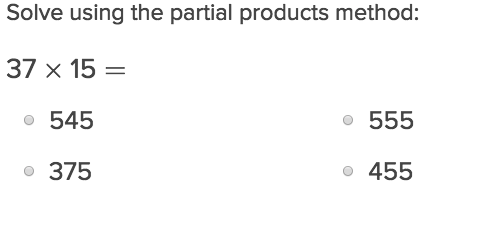Multi-Digit Multiplication and Partial Products 2
Exercise
Multi-Digit Multiplication and Partial Products 2
For students just learning how to deal with partial products, this exercise will make the job less complicated.
Math
Exercise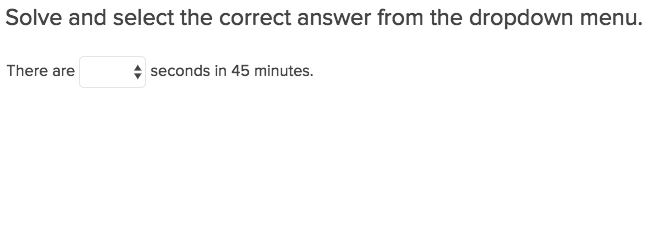Multi-Digit Multiplication and Word Problems 1
Exercise
Multi-Digit Multiplication and Word Problems 1
Once students have understood the basics of multi digit multiplication word problems, set them to task on this exercise that will bring it all full circle.